# The Number Hiding Inside the Spirograph

A Part 2 has been created that explores the number introduced below in greater detail.

A few weeks ago I was casually cleaning up my personal site and out of curiosity I decided to take a look at an old spirograph simulation I wrote back in 2009. What was supposed to be 5-10 minutes of reading turned into a few hours of adding obvious improvements that I somehow missed the first time around.

While playing around with the new code I noticed that a special value for one of the parameters would result in a spirograph where each of the "petals" were tangent to each other: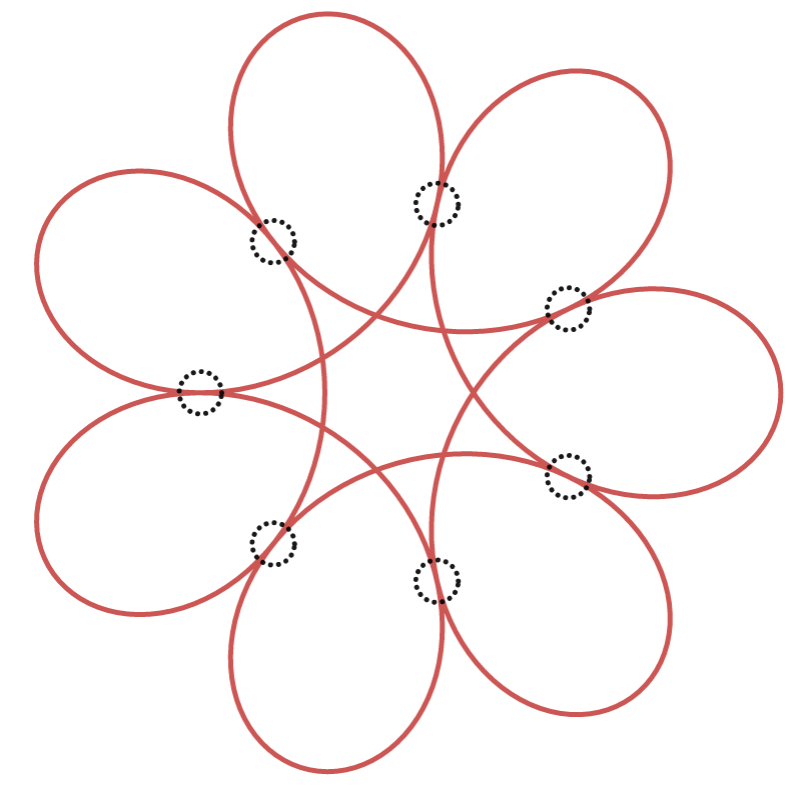I also noticed that as the number of the petals increased, that special value tended to a specific number. That piqued my interest, what was that number?

## The Spirograph Function

Before we can solve for that number, we need to introduce the function that describes the path of the spirograph. The output of this function is a complex number that represents the 2D location of the spirograph pen:

is the ratio of the "pen" radius to the inner circle radius. is the ratio of the outer circle radius to the inner circle radius subtracted by one. is the angular position of the inner circle relative to the origin. The following image should help visualize what these parameters mean:Geometrically the parameter dictates the shape of the spirograph and the parameter dictates the number of petals the spirograph has. I've created an interactive graph that you can use to get a good understanding of the relationship between theses parameters and the shape of the spirograph.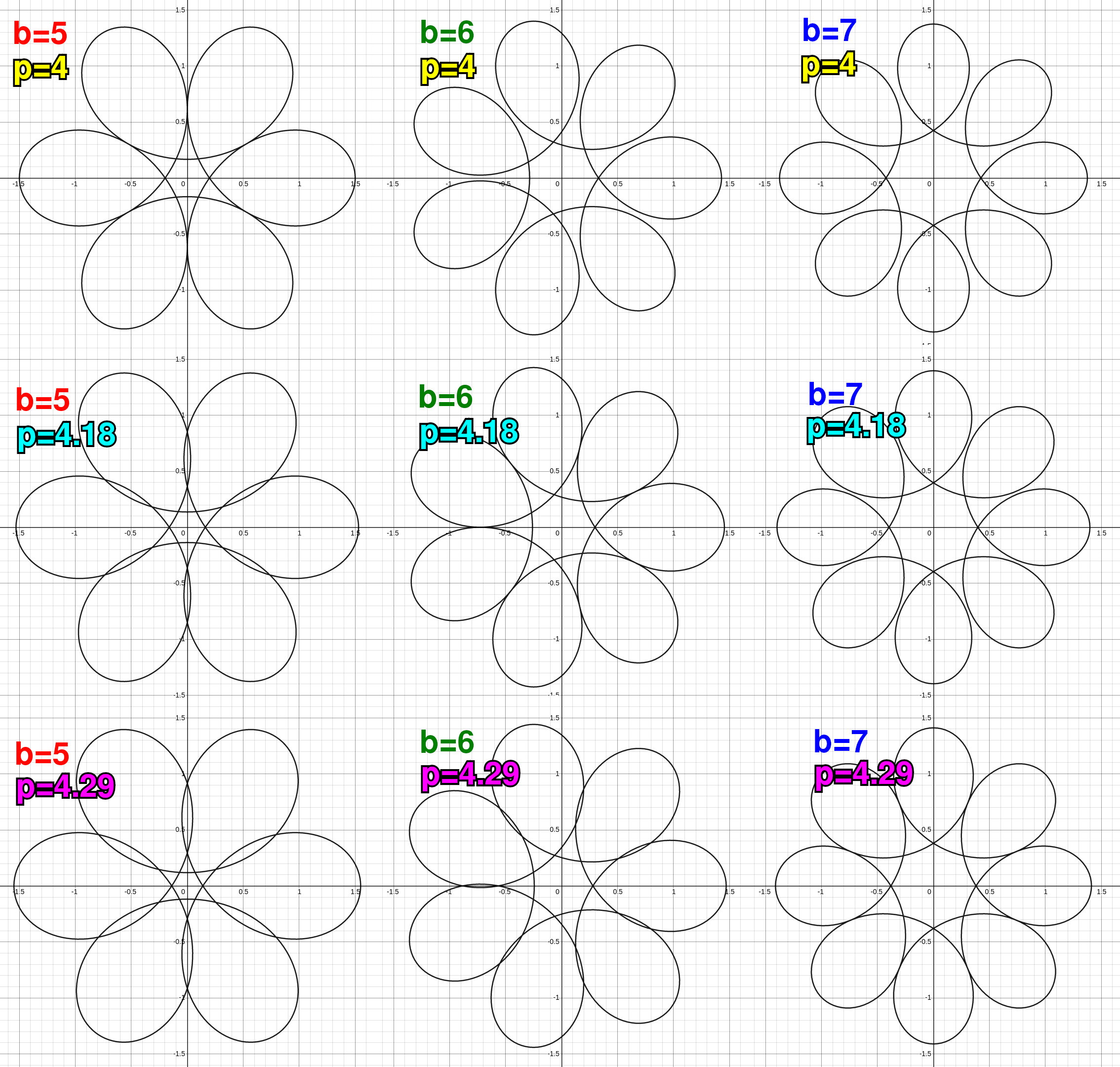You'll notice that for each value in the preceding image there is a specific value that causes each petal to be tangent to the next one. As grows indefinitely, the value of that causes each petal to be tangent to the next one tends towards a specific number. That's the number we want to solve for.

Before we attempt our solution we should enumerate general properties of the spirograph function. These will be properties that hold true for all values of , , and .

The spirograph is symmetric across the x-axis. This means its complex conjugate is equal to the output value at the negative input:

The spirograph also has rotational symmetry every radians:

Since these properties apply regardless of the parameters of the function, we know to avoid them when specifying constraints relevant to our solution.

While we're at it, let's introduce the derivative of the spirograph function since we will also use it to specify constraints relevant to our solution:

## The Constraints

Our ultimate goal is to find the value of as such that the petals of the spirograph are tangent to each other. Our strategy will be to first try to solve for that special in terms of and then try to take the limit of that expression as . To do that, we will apply algebraic constraints to the spirograph function that only apply when the spirograph meets certain geometric criteria.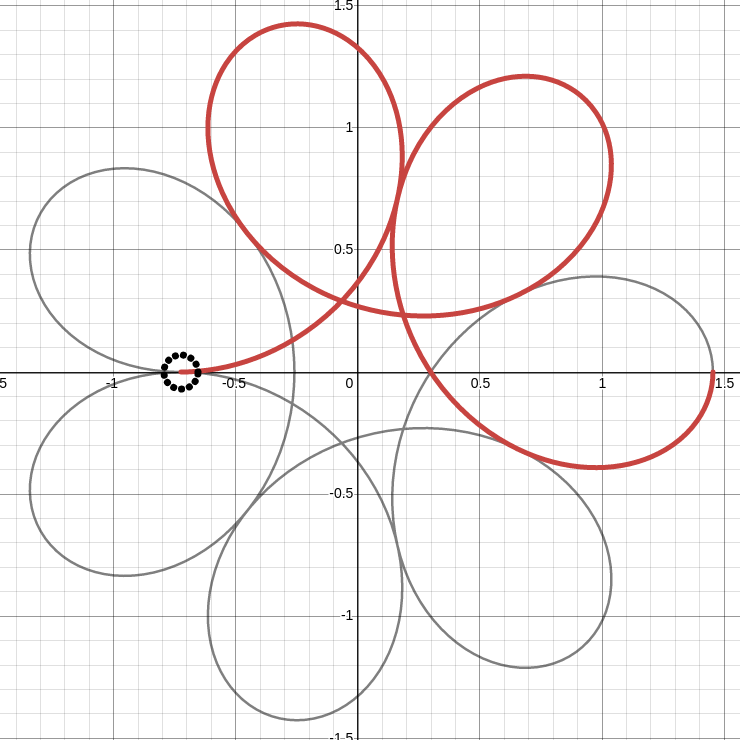From the image above we can see that when the spirograph petals are all tangent to each other, there is a specific input value such that the spirograph is tangent to the x-axis and its neighboring petal. This means that the function will have a zero imaginary component (no vertical offset) at :

The tangency at the x-axis also means that the velocity vector (the derivative of ) will have a zero imaginary component at :

We have two equations and two unknowns: and . We can combine and to remove and get an equation in terms of alone.

At this point we have reduced our constraints to one equation in . The problem is that there is no trivial way to solve for in these terms. We have three options:

1. Treat and as distinct indeterminates and use another constraint to solve for them separately.
2. Put in terms of and then solve for . This can be done relatively easily when is a positive integer, which is true in this case.
3. Solve for numerically.

Let's explore each option separately.

## Solving for tanω and tan(bω) separately

If we want to treat and as distinct indeterminates so that we can solve for them separately we need another constraint. The natural thing to do would be to employ constraints on the real components of and at , which we will respectively call and .

Then we replace using :

The preceding equation gives us an expression for but we are looking for an expression for , so let's try applying a constraint on the real component of .

Replace using :

We can combine and to get a new expression for :

Since we have an equation for , let's put in terms of . We'll use the identity and :

Replace using :

Replace using :

We only really care about the value of as . Geometrically it can be inferred that . If we assume that exists then we can combine that information to get the following expression for as :

This is where we get stuck. We don't have a new independent expression for , in general or as . Without more constraints the problem of finding as is equivalent to the problem of finding as .

## Rewriting tan(bω) in terms of tan(ω)

When you constrain to be a positive integer, it turns out that there is a way to express in terms of :

Using this identity for we can then solve for . Unfortunately this will only work for finite values of in theory and only sufficiently small finite values of in practice. So it won't work for solving for as but it's an interesting outcome anyway and it gives deeper insight into the problem.

Replace with using :

To go further we'll have to assume that is either even or odd. The answer works out the same in either case. For this derivation we'll assume is odd. We'll leave the derivation when assuming is even as an exercise for the reader. When is odd, then and .

Now let's simplify the coefficient:

Now replace the new expression for the coefficient back into the summation. Also replace summation bounds with to accomodate for the case when is even:

This equation looks neat and elegant but our goal is to solve for not . Let's use to get in terms of :

We can replace this expression for into so we can solve for directly in the summation:

Ideally the summation would be in a form where each power of appears in only a single summation term:

Keeping with our goal of getting the summation into a form where each power of the variable appears in a only a single summation term, we have to rearrange the sum so that the outer summation is over (this step is non-intuitive):

Finally we have derived a polynomial in that we can plug into a solver to get its exact solution. Since the degree of this polynomial is , by the fundamental theorem of algebra, that means there are up to distinct solutions for , not just one. What are these other solutions? For there are 3 solutions, here they are: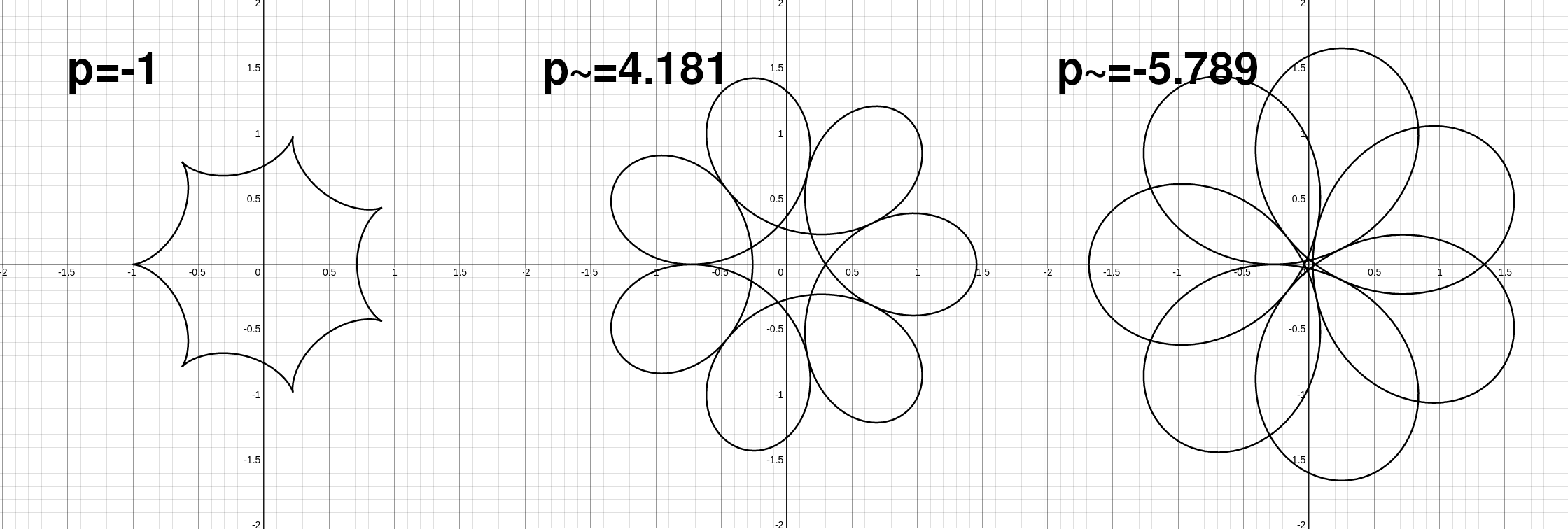You can see that each of the solutions above satisfy the constraints we specified yet we were only looking for the second solution. This shows that our constraints are not specific enough. Are there other algebraically convenient constraints that could be used to isolate the solution we want?

Since the we are looking for occurs as and our polynomial is of degree that implies it's the solution of a polynomial of infinite degree, hinting that is potentially a transcendental number, i.e. to express it in exact form using only integers and primitive operations would require infinite terms. Can it be proven to be a transcendental number as ?

Here are the exact solutions for the first few values of . Do you see a pattern?

## Solving for ω Numerically

So far we've been able to derive interesting information about but getting an exact expression for as has eluded us. That doesn't mean we're not able to approximate it numerically. Let's look at the graph of (from ):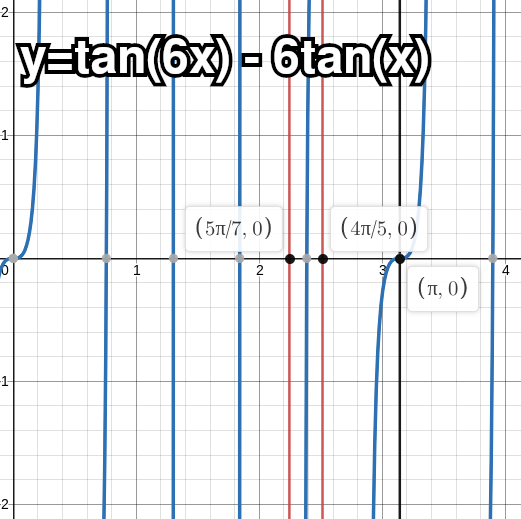The solutions for that satisfy our constraints are the points where the graph intersects the x-axis. The careful observer will notice that the specific solution for which we are looking lies in the interval . Additionally the function is increasing on this interval. This allows us to write a relatively simple binary search algorithm to approximate our solution:


#include <stdio.h>
#include <math.h>
#include <limits.h>

int main() {
unsigned long b;
double plast = 0;
double pi = atan(1) * 4;

for (b = 4; ; b *= 2) {
double lo = pi * (b - 1) / (b + 1);
double hi = pi * (b - 2) / (b - 1);
double last = lo;
double p;

while (1) {
double mid = (lo + hi) / 2;

/* root has not changed, we are as close as possible */
if (mid == last) {
break;
}

if (tan(b * mid) > b * tan(mid)) {
hi = mid;
} else {
lo = mid;
}

last = mid;
}

p = cos(last) / cos(b * last);

/* as b->oo, (lo+hi)/2 approaches pi, if last is not
able to hold enough precision to differentiate it
from pi, then cos(last) == -1, thus reaching our
limit of precision */
if (p < 0) {
break;
}

if (p == plast) {
/* p didn't change across iterations, we've reached limit
of precision */
break;
}

plast = p;

if (b - 1 == ULONG_MAX) {
break;
}
}

printf("p = %.9f (at b = %lu)\n", plast, b);

return 0;
}


On my machine this prints p = 4.603338955 (at b = 134217728).

## Conclusion

While we weren't able to derive an exact expression for we were able to discover some of its properties and a basic numerical algorithm to approximate it. Some questions remain: Is a transcendental number as ? More generally, for what values of is a transcendental number? How about ? If and when and/or are transcendental numbers, are they finite functions of known transcendental numbers like and ? More clever math is required to answer these questions, until then we may never know.

As we saw, given the constraints we used, doesn't have a single solution. There are an infinite amount of related numbers that correspond to the spirograph petals being tangent to each other at different intervals. I call these numbers the Petal Numbers. Is there a deeper significance to these numbers? I'm not sure yet. I may revisit this problem in the coming years as my abilities in math continue to mature.

Rian Hunter, 6/11/21

## Update!

6/12/21: Reddit user existentialpenguin found that our computed approximate number matches up very closely with a number already documented in the OEIS though with a seemingly different formulation. Our as can be expressed as a solution to in the interval . Following the references in the OEIS entry, I also found that as is a close match to another number documented in the OEIS: it can be expressed as the solution to in the interval , i.e. it's a fixed point of the tangent function. The potential correspondence between the spirograph problem and these problems may be explored in a future article.

6/16/21: There is now a Part 2 that finds an exact expression for , proves that it's transcendental, and provides an efficient way to compute .